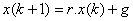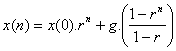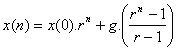## Mixed Arithmetic and Geometric Growth

Difference equation :Functional shortcut:orCharacteristics:

• Multiply by a constant growth factor then add a constant

Try your own input to the interactive program of Mixed Arithmetic and Geometric Growth Model below. Input the initial value x(0) and constant r and g then click "Find" button to find x(n) and to show the plot.

Initial value x(0) = Constant r = > 0 (must be positive) Constant g = Find the value of x at n =

Preferable reference for this tutorial is

Teknomo, Kardi (2015) Simple Growth Model. https:\\people.revoledu.com\kardi\ tutorial\Growth\